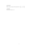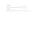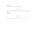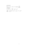MA17xexam00pdfLoading previews...
 first order linear differential equation Text Download (13kB)first order linear differential equationorder of a matrixdeterminant of a matrixsimultaneous equationspartial differentiation
32 files in this resource

MA17xexam00pdf

Exam questions and solutions in PDFView Item